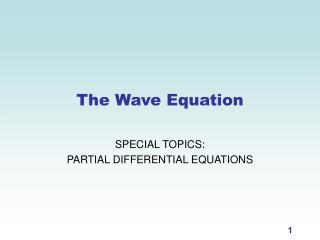# The Wave Equation - PowerPoint PPT PresentationDownload PresentationThe Wave Equation

The Wave Equation
Download Presentation## The Wave Equation

- - - - - - - - - - - - - - - - - - - - - - - - - - - E N D - - - - - - - - - - - - - - - - - - - - - - - - - - -
##### Presentation Transcript

1. The Wave Equation SPECIAL TOPICS: PARTIAL DIFFERENTIAL EQUATIONS

2. Motion of a string Imagine that a stretched string is vibrating. The wave equation says that, at any position on the string, acceleration in the direction perpendicular to the string is proportional to the curvature of the string.

3. The one-dimensional wave equation Let • x = position on the string • t = time • u(x, t) = displacement of the string at position x and time t. • T = tension (parameter) •  = mass per unit length (parameter) Then Equivalently,

4. Solving the one-dimensional WE First, we make a wild assumption: suppose that u is a product of a function of x and a function of t: Then the wave equation becomes So, The only way for this equation to be true for allx and for all t is for both sides to be constant; that is, T’’(t)/a2T(t) = and X”(x)/X(x) =.

5. Separation of variables Now means that If  < 0,T(t)and X(x) are both trig functions.* *If  = 0, they’re linear, and if  > 0, they’re exponential. But let’s not worry about that now. (Explanation on next slide.)

6. Clarification: the sign of  There are three types of solutions to the equation • if  = 0, then X(x) is linear (ax + b), which won't satisfy the boundary conditions; • if  > 0, X(x) is exponential (ket), which also won't satisfy the boundary conditions; and • if  < 0, then X(x) is a sinusoid that satisfies the boundary conditions. This is why in slide 8.

7. 0 L Boundary conditions In a stringed instrument, each end of the string is fixed; if the string has length L, then, for all t, Since T(t) ≠ 0 for all t, X(0) = X(L) = 0; thus, X(x) could be a sum of sine terms with zeros at x = L:

8. Finding T(t) Now we know two things: This means that Now substitute  into the equation for T: And find the solution: The minus sign is correct. The original slide was wrong.

9. Putting it all together We now have a family of solutions for the wave equation (one for every n): Suppose we choose an x0 and look at how the displacement varies at this point as t changes. This is the equation of a sinusoid with frequency = where n = 1, 2, 3…

10. Increase n (discrete changes) Overblowing Playing harmonics Increase a (continuous changes) Tuning Decrease L (continuous changes) Shortening Fretting Take it higher So there are three ways to increase the frequency of a sound, producing a “higher” note:

11. Boundary conditions With wind instruments, it’s not always true that if L is the length of the tube, u(0, t) = u(L, t) = 0. This is true with the flute (because the pressure doesn’t change at the ends). However, what happens with some instruments (like the sax) is that ux(0, t) = ux(L, t) = 0, which means that the X(x) functions are cosine terms rather than sines. Still more bizarre is the behavior of the clarinet. It has boundary conditions Go back and substitute in these boundary conditions to see what happens!

12. Standing waves The harmonics we hear on a stringed instrument and the overtones on a wind instrument are actually produced by standing waves, which are solutions of the form Sketch some standing waves (see demo on the web). How are the standing waves for a clarinet different from those for a flute?

13. Exercises • Verify that the sum of any two solutions to the wave equation is also a solution. • Redo the wave equation solution using the boundary conditions for a flute: ux(0, t) = ux(L, t) = 0 • Redo the wave equation solution using the boundary conditions for a clarinet: u(0, t) = ux(L, t) = 0. Find the frequencies of the solutions, and sketch the standing waves that are solutions to this equation. • Use separation of variables to find a family of solutions to the heat equation: with boundary conditions u(0, t) = u(L, t) = 0.